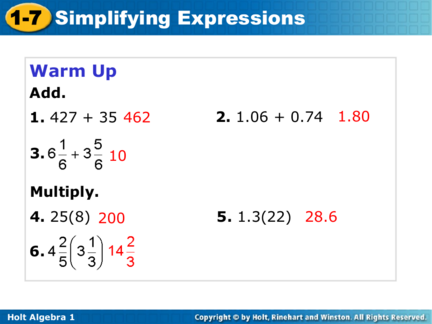# Simplification of Algebraic ExpressionsContributed by:This pdf contains the following topics:-
Distributive property
Coefficient
Combining Like Terms
Simplify by combining like terms.
Examples and many more
1. 1-7 Simplifying Expressions
Warm Up
1. 427 + 35 462 2. 1.06 + 0.74 1.80
3. 10
Multiply.
4. 25(8) 200 5. 1.3(22) 28.6
6.
Holt Algebra 1
2. 1-7 Simplifying Expressions
The Distributive Property is used with Addition to
Simplify Expressions.
The Distributive Property also works with
subtraction because subtraction is the same as
Holt Algebra 1
3. 1-7 Simplifying Expressions
The terms of an expression are the parts to be
added or subtracted. Like terms are terms that
contain the same variables raised to the same
powers. Constants are also like terms.
Like terms Constant
4x – 3x + 2
Holt Algebra 1
4. 1-7 Simplifying Expressions
A coefficient is a number multiplied by a
variable. Like terms can have different
coefficients. A variable written without a
coefficient has a coefficient of 1.
Coefficients
1x2 + 3x
Holt Algebra 1
5. 1-7 Simplifying Expressions
combine like terms. You can factor out the
common factors to simplify the expression.
7x2 – 4x2 = (7 – 4)x2 Factor out x2 from both terms.
= (3)x2 Perform operations in
parenthesis.
= 3x2
Notice that you can combine like terms by
adding or subtracting the coefficients and
keeping the variables and exponents the same.
Holt Algebra 1
6. 1-7 Simplifying Expressions
Example 3A: Combining Like Terms
Simplify the expression by combining like
terms.
72p – 25p
72p – 25p 72p and 25p are like terms.
47p Subtract the coefficients.
Holt Algebra 1
7. 1-7 Simplifying Expressions
Example 3B: Combining Like Terms
Simplify the expression by combining like
terms.
A variable without a coefficient
has a coefficient of 1.
and are like terms.
Write 1 as .
Holt Algebra 1
8. 1-7 Simplifying Expressions
Example 3C: Combining Like Terms
Simplify the expression by combining like
terms.
0.5m + 2.5n
0.5m + 2.5n 0.5m and 2.5n are not like terms.
0.5m + 2.5n Do not combine the terms.
Holt Algebra 1
9. 1-7 Simplifying Expressions
Check It Out! Example 3
Simplify by combining like terms.
3a. 16p + 84p
16p + 84p 16p + 84p are like terms.
3b. –20t – 8.5t2
–20t – 8.5t2 20t and 8.5t2 are not like terms.
–20t – 8.5t2 Do not combine the terms.
3c. 3m2 + m3
3m2 + m3 3m2 and m3 are not like terms.
3m2 + m3 Do not combine the terms.
Holt Algebra 1
10. 1-7 Simplifying Expressions
Example 4: Simplifying Algebraic Expressions
Simplify 14x + 4(2 + x). Justify each step.
Procedure Justification
1. 14x + 4(2 + x)
2. 14x + 4(2) + 4(x) Distributive Property
3. 14x + 8 + 4x Multiply.
4. 14x + 4x + 8 Commutative Property
5. (14x + 4x) + 8 Associative Property
6. 18x + 8 Combine like terms.
Holt Algebra 1
11. 1-7 Simplifying Expressions
Check It Out! Example 4a
Simplify 6(x – 4) + 9. Justify each step.
Procedure Justification
1. 6(x – 4) + 9
2. 6(x) – 6(4) + 9 Distributive Property
3. 6x – 24 + 9 Multiply.
Combine like terms.
4. 6x – 15
Holt Algebra 1
12. 1-7 Simplifying Expressions
Check It Out! Example 4b
Simplify −12x – 5x + 3a + x. Justify each step.
Procedure Justification
1. –12x – 5x + 3a + x
2. –12x – 5x + x + 3a Commutative Property
3. –16x + 3a Combine like terms.
Holt Algebra 1
13. 1-7 Simplifying Expressions
Lesson Quiz: Part II
Simplify each expression by combining like
terms. Justify each step with an operation or
property.
5.
6. 14c2 – 9c 14c2 – 9c
7. 301x – x 300x
8. 24a + b2 + 3a + 2b2 27a + 3b2
Holt Algebra 1